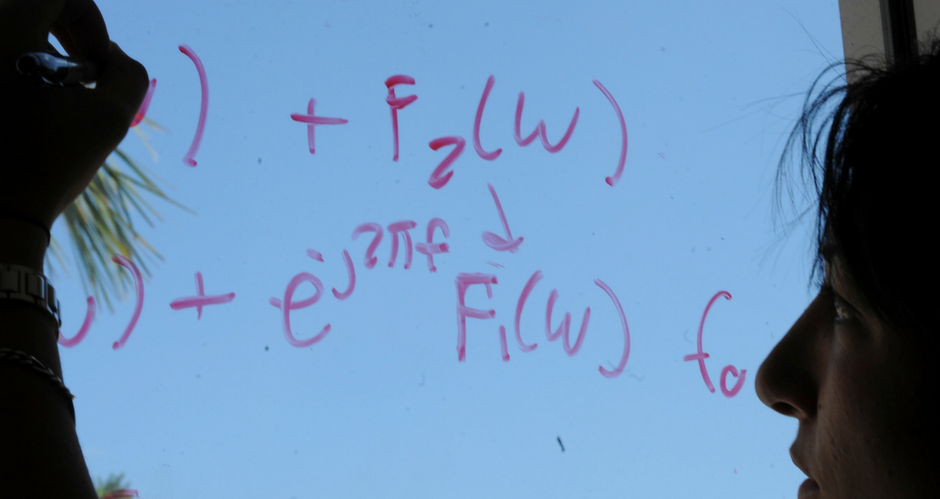## Mathematics and System Engineering Faculty Publications

### Existence of a Solution of a Fourier Nonlocal Quasilinear Parabolic Problem

Article

#### Publication Title

Journal of Applied Mathematics and Stochastic Analysis

#### Abstract

The aim of this paper is to give a theorem about the existence of a classical solution of a Fourier third nonlocal quasilinear parabolic problem. To prove this theorem, Schauder’s theorem is used. The paper is a continuation of papers [l]- and the generalizations of some results from -. The theorem established in this paper can be applied to describe some phenomena in the theories of diffusion and heat conduction with better effects than the analogous classical theorem about the existence of a solution of the Fourier third quasilinear parabolic problem.

43

67

#### DOI

10.1155/S1048953392000042

1992

COinS